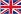English

## PRODUCTS

You are here: » » » » volumn Flowmeter# volumn Flowmeter

The flow rate is the ratio of the amount of fluid flowing through the cross section of the pipe to the time taken for that amount to pass through the section.

What is flow?

The flow rate is the ratio of the amount of fluid flowing through the cross section of the pipe to the time taken for that amount to pass through the section. The flow is divided into volume flow and mass flow.

What is volume flow?

Volume flow refers to the amount of fluid expressed in volume.

The common flow measurement for the volume contains orifice plate, turbine flow meter, vortex flow meter, electromagnetic flow meter, rotary flow meter, ultrasonic flow meter and elliptical gear flow meter.

What is mass flow?

Mass flow rate refers to the flow rate of fluid quantity expressed by mass.

What is flow?

The flow rate is the ratio of the amount of fluid flowing through the cross section of the pipe to the time taken for that amount to pass through the section. The flow is divided into volume flow and mass flow.

What is volume flow?

Volume flow refers to the amount of fluid expressed in volume.

The common flow measurement for the volume contains orifice plate, turbine flow meter, vortex flow meter, electromagnetic flow meter, rotary flow meter, ultrasonic flow meter and elliptical gear flow meter.

What is mass flow?

Mass flow rate refers to the flow rate of fluid quantity expressed by mass.

Previous:
Next:

## Get In Touch

Sales Department: